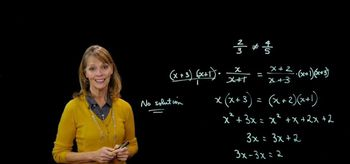Start typing, then use the up and down arrows to select an option from the list.
2:56 minutes
Problem 85
Textbook Question

# The equations in Exercises 79–90 combine the types of equations we have discussed in this section. Solve each equation. Then state whether the equation is an identity, a conditional equation, or an inconsistent equation. 2/x + 1/2 = 3/4Verified Solution
This video solution was recommended by our tutors as helpful for the problem above.
25views